Courses
Courses for Kids
Free study material
Free LIVE classes
More

# Play with Patterns Class 4 Notes CBSE Maths Chapter 10 (Free PDF Download)LIVE
Join Vedantu’s FREE Mastercalss

## Learn Recognising and Drawing Patterns with Class 4 Maths Chapter 10 Revision Notes

You have learnt different kinds of patterns and how to recognise them in the previous classes. The Class 4 Maths syllabus offers another chapter in this context to teach students advanced concepts of pattern recognition and making. Studying Play with Patterns will be a fascinating part of your curriculum.

Download and refer to the Play with Patterns revision notes to understand the concepts of patterns easily. The experts have explained them in easy to comprehend language. You can also practice the worksheets and evaluate your skills related to patterns.

Last updated date: 29th Sep 2023
Total views: 145.8k
Views today: 1.45k

## Access Class - 4 Mathematics Chapter - 10 Play with Patterns Revision Notes

### Pattern

• A pattern is referred to as the arrangement of shapes, numbers, and colours which are in repetition.

• In Mathematics, the sequence is sometimes called a pattern.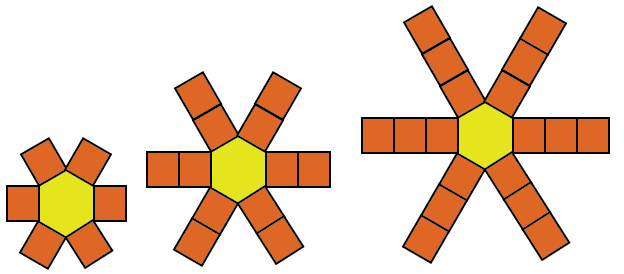Image: Pattern

### Different Types of Patterns

• Repeating Pattern: If any event or object keeps repeating itself over and over then the pattern is called a Repeating Pattern.

• In the below figure, after every red block two blue blocks are repeated. Hence it follows the Repeating Pattern.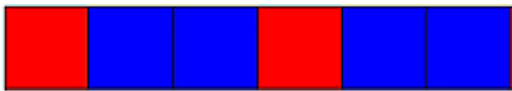Image: Repeating Pattern

• Growing Pattern: If any event or object keeps growing regularly then formed is called a Growing Pattern.

• In the below figure, one block is added at each site. Hence, the figure follows a Growing Pattern.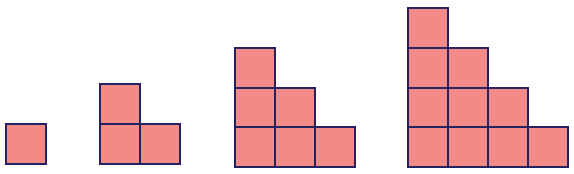Image: Growing pattern

• Shrinking Pattern: If any event or object keeps decreasing in a regular interval then the pattern is called a Shrinking Pattern.

• In the below figure, two blocks are diminishing at each step. Hence the below figure follows a shrinking pattern.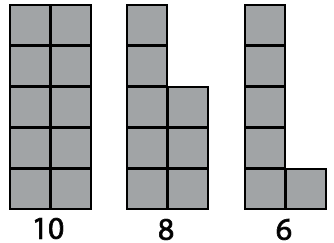Image: Shrinking Pattern

### Solved Examples

Q1. Complete the following pattern?Ans: The above figure shows clearly that it is a repeating pattern.

• The first two figures have capital letters A and then the third place contains letters as capital B. Again in the fourth and fifth places the letters contained are capital A and in the sixth place, the letter is capital B.

• Similarily to repeating the pattern the seventh and eighth places will also contain letters as capital A  and the eighth place would be capital B. The complete pattern is given belowQ2. Complete the following pattern?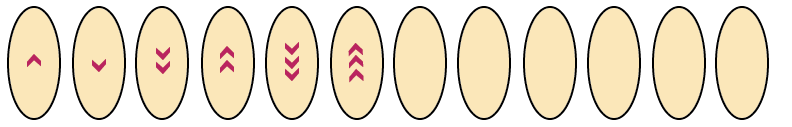Image: Growing Pattern

Ans: In the above-given figure, it is clear that the pattern involved in the Growing Pattern.

• In each consecutive figure the arrows are increasing by one in both upward and downward directions.

• In the first figure, there is one arrow pointing in an upward direction, in the second figure the arrow is downwards pointing in the downward direction.

• Similarly in the third and fourth figures the arrows pointed are two upward and downwards respectively, and for ffiguresfifth and sixth the arrows are three upward and downward respectively.

• Now for the seventh and eighth figures, the arrows will be four upward and downward respectively.

• Similarly we can calculate arrows for the rest of the vacant figures.

The complete pattern is given below.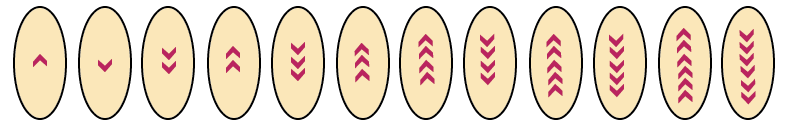Image: Growing pattern in form of an arrow sign

### Number Patterns

A number Pattern is referred to as the sequence followed by a certain group of numbers. For example even number patterns and odd number patterns.

• Even Number Patterns: A number pattern followed by a list of even numbers is called an Even Number Pattern. For example : 4,6,8,10,12,14,

• Odd Number Patterns: A number pattern followed by a list of odd numbers is called an odd  Number Pattern.  For example : 3,5,7,9,11,13,.........

• Growing Number Patterns: A number pattern that increases or decreases by content digit /unit then the pattern formed is referred to as the Growing Number Pattern. For example4,8,12,16,20,........ (here it is growing by adding 4 numbers).

### Solved Examples

Complete the following number patterns?

Q1. 3, 6, 10, __, __, __

Ans. First Digit = 3

Second Digit = 3 + 3 = 6

Third Digit = 6 + 4 = 10

We will add 5,6, and 7 to the preceding digits respectively to find the rest of the digits.

Fourth Digit = 10 + 5 = 15

Similarly , Fifth Digit = 15 + 6 = 21

Sixth Digit = 21 + 7 = 28

Hence the pattern is 3,6,10,15,21,28

Q2. 2, 6, 12, __, __, __

Ans. First Digit = 2

Second Digit = 2 + 4 = 6

Third Digit = 6 + 6 = 12

We will add 8,10, and 12 to the preceding digits respectively to find the rest of the digits.

Fourth Digit = 12 + 8 = 20

Similarly , Fifth Digit = 20 + 10 = 30

Sixth Digit = 30 + 12 = 42

Hence the pattern is 2, 6, 12, 20, 30, 42

Q3. 6, 11, 18, __, __, __

Ans. First Digit = 6

Second Digit = 6 + 5 = 11

Third Digit = 11 + 7 = 18

We will add 9,11, and 13 to the preceding digits respectively to find the rest of the digits.

Fourth Digit = 18 + 9 = 27

Similarly , Fifth Digit = 27 + 11 = 38

Sixth Digit = 38 + 13 = 51

Hence the pattern is 6, 11, 18, 27, 38, 51

### Secret Messages

• Secret Messages are referred to as messages contained in the form of numbers and alphabets.

• By using the alphabet and numbers a secret message can be sent from one person to another person.

• To decode the secret messages, we will always separate alphabets and numbers.

### Names in an Order

• Names are always arranged in alphabetical order.

• Names which are pronounced the same from both rights and left are referred to as special Names. For example Naman.

### Solved Example

Q1. Decode the below-given message?

I1C2A3N4D5R6I7V8E

Ans1: We have I1C2A3N4D5R6I7V8E, to decode this secret message we will separate alphabets and numbers, we get  I CAN DRIVE. Therefore, the secret message is I CAN DRIVE.

Q2.Write an example of a special name?

Ans2. We know that special names are pronounced the same from both right and left. For example, Kanak, here if we read Kanak from either left or right, the name would be pronounced the same.

### Practise Problems

Q1. Complete the following given number patterns?

(a) 98, __, 102, __, __, __

(b) 3, 7, 13, __, __

Ans: (a) 98, 100, 102, 104, 106, 108

(b) 3, 7, 13, 21, 31, 43

Q2. Decode the below-given message?

(a) I1w2I3L4L5G6o

(b) A1R2E3Y4O5U6M7A8D

Ans2:  (a) I WILL GO

## Importance of CBSE Class 4 Chapter 10 Play with Patterns

Take a look at these points to understand the importance of Maths Chapter 10 for students of Class 4.

• Students will learn how to recognise complex patterns. They will check the image illustrations to recognise the patterns and will answer the question by using logic.

• This chapter is not associated with the common mathematical operations conducted in other chapters. It is all about creating patterns by rearranging elements provided in the problems. If you check the Play with Patterns summary, you will understand that the prime objective of this chapter is to develop pattern recognition and development skills in young minds.

• On progressing further, the different methods of addition and subtraction will be introduced for creating and recognising patterns.

• The chapter also has questions in puzzle formats. Solving such questions will help you to study pattern for Class 4 and develop advanced ideas.

• The best examples of number patterns given in the exercise problems will surely intrigue your mind to learn more. You will find patterns in numbers explained well. The concepts will become clearer when you follow the revision notes.

## Benefits of Vedantu’s Play with Patterns Class 4 Worksheets with Answers and Revision Notes

• Simpler elaboration and explanation of the concepts will help you understand them easily.

• Faster turnaround when you are preparing for an exam

• Use the worksheets to practice and become better at solving these problems

• Refer to the solutions of patterns in Maths for Class 4 so that you can understand and adopt the techniques shown by the experts

• Recall the studied concepts, principles, and techniques before an exam using the revision notes

Download the PDF files of revision notes and worksheets for free. Practice solving the problems and develop excellent pattern recognition skills. Find out patterns around us and develop confidence by following the revision notes.

Download the NCERT solutions to become better at solving questions related to geometry and patterns for Class 4. Refer to these notes and solutions at your convenience and resolve your queries on your own. Stay ahead of the curve by preparing well before an exam using these resources.

## FAQs on Play with Patterns Class 4 Notes CBSE Maths Chapter 10 (Free PDF Download)

1. How can you find a pattern in a set of numbers?

Check whether these numbers add or subtract to form something similar or not. You can also try other mathematical operations to check the same. Follow the clues given in a problem to find patterns.

2. How can you prepare Chapter 10 Patterns well?

Follow the classroom sessions, use the revision notes for clarifying your doubts, and solve the exercise questions. Proceed to solve the worksheet provided with the notes to become more skilled at recognizing patterns.

3. Why should I refer to the solutions given?

The solutions are compiled by the experts following Class 4 CBSE standards. These solutions will help you find out the right format to follow for answering questions methodically to score better in exams.